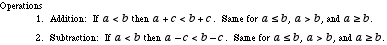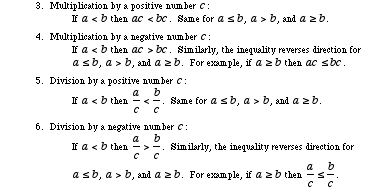index: click on a letter A B C D E F G H I J K L M N O P Q R S T U V W X Y Z A to Z index index: subject areas numbers & symbols sets, logic, proofs geometry algebra trigonometry advanced algebra & pre-calculus calculus advanced topics probability & statistics real world applications multimedia entrieswww.mathwords.com about mathwords website feedback

 Inequality Rules Algebra rules for manipulating inequalities are listed below.Transitive Property of Inequalities If a < b and b < c , then a < c. If a ≤ b and b ≤ c , then a ≤ c. If a > b and b > c , then a > c. If a ≥ b and b ≥ c , then a ≥ c.   See also